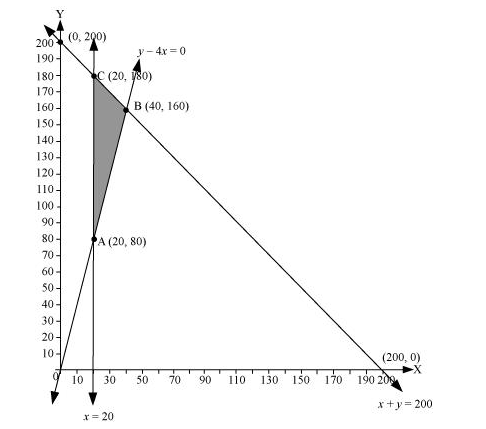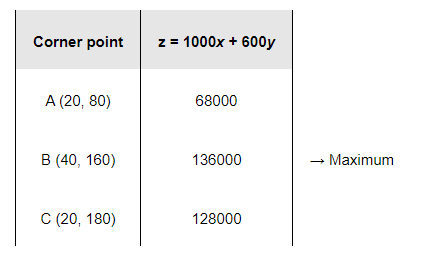# An aeroplane can carry a maximum of 200 passengers.

Question:

An aeroplane can carry a maximum of 200 passengers. A profit of Rs 1000 is made on each executive class ticket and a profit of Rs 600 is made on each economy class ticket. The airline reserves at least 20 seats for executive class. However, at least 4 times as many passengers prefer to travel by economy class than by the executive class. Determine how many tickets of each type must be sold in order to maximize the profit for the airline. What is the maximum profit?

Solution:

Let the airline sell tickets of executive class and y tickets of economy class.

The mathematical formulation of the given problem is as follows.

Maximize $z=1000 x+600 y$                                   (1)

subject to the constraints,

$x+y \leq 200$                             (2)

$x \geq 20$                                  (3)

$y-4 x \geq 0$                              (4)

$x, y \geq 0$                                (5)

The feasible region determined by the constraints is as follows.The corner points of the feasible region are A (20, 80), B (40, 160), and C (20, 180).

The values of z at these corner points are as follows.The maximum value of z is 136000 at (40, 160).

Thus, 40 tickets of executive class and 160 tickets of economy class should be sold to maximize the profit and the maximum profit is Rs 136000.